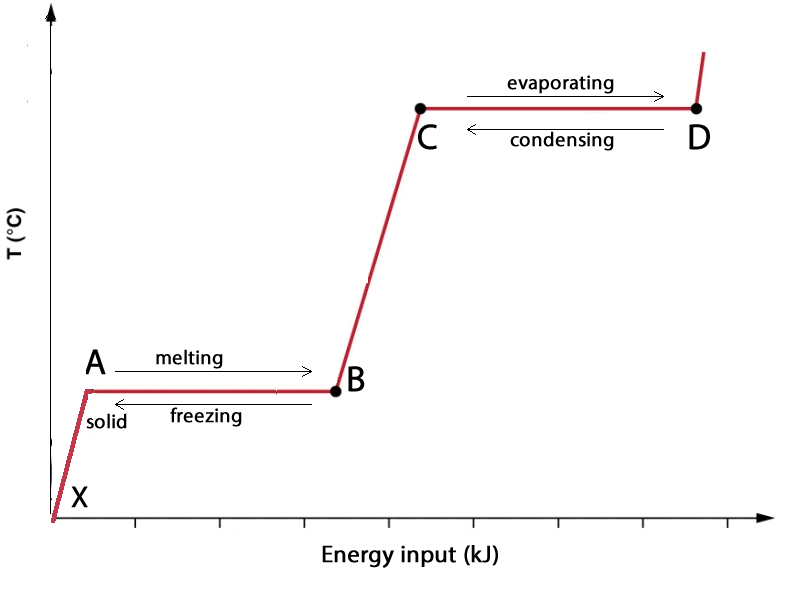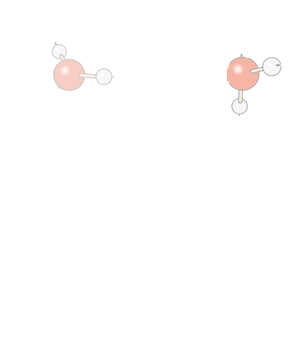Phase change diagramsWhen a substance changes state, for example, as it changes from a solid to a liquid or liquid to gas, it requires energy to undergo this change. When the revers happens, for example, when a substance changes form gas to a liquid it requires energy to be lost in order to undergo this change is state. If we plot the temperature change versus heat energy supplied for a solid substance we get a graph similar in shape to the one above. At point A all particles are in the solid state. As we move across from A to B the number of particles found in the liquid state increase until at B all the substance is in the liquid state. From C to D the substance gradually changes to the gas state until at D all the substance is in the gaseous state. At point A the substance has just reached its melting temperature but it is still in the solid state. As it now progresses through to point B more and more particles will transition to the liquid state where at point B the entire substance is a liquid. At point C the substance has just reached its boiling temperature but remains in the liquid state, as it progresses over to point D more and more particles transition to the gaseous state until the entire substance is a gas at D. Notice at regions A-B and C-D the temperature remains constant. While at regions X-A and B-C the temperature of the substance gradually increases. These regions tell us something about what is happening to the energy being supplied. Now consider that temperature is an indicator of the average kinetic energy of the particles of the substance whose temperature is being measured. Two points to consider. 1. In regions X-A and B-C the energy is increasing the average kinetic energy of the particles of the substance. That is, the average speed of the particles is increasing. 2. Regions A-B and C-D represent the energy required to change the state of a given amount of substance and is known as the latent heat. Depending on which state change is taking place the latent heat has a particular name. For example: - solid -> liquid = Latent heat of fusion (input of energy) - liquid -> gas = Latent heat of vaporisation (input of energy) - gas-> liquid = Latent heat of condensation (energy given out) - liquid -> solid = Latent heat of freezing (energy given out) 3. For any substance the: - magnitude of the latent heat of fusion = the magnitude of the latent heat of freezing. - magnitude of the latent heat of vaporisation = the magnitude of the latent heat of condensation. Let's look at water as an example.